# Get college algebra help

Recent questions in College algebraGabriella Peterson 2022-08-28

2022-08-27

### How do you convert 2.8 (8 being repeated) to a fraction?nainamourya181 2022-08-25

### If (R, +, .) Is a ring and a belongs to R then S = { x belongs to R : ax = 0} is a subring of RFeranmimakun6 2022-08-25

###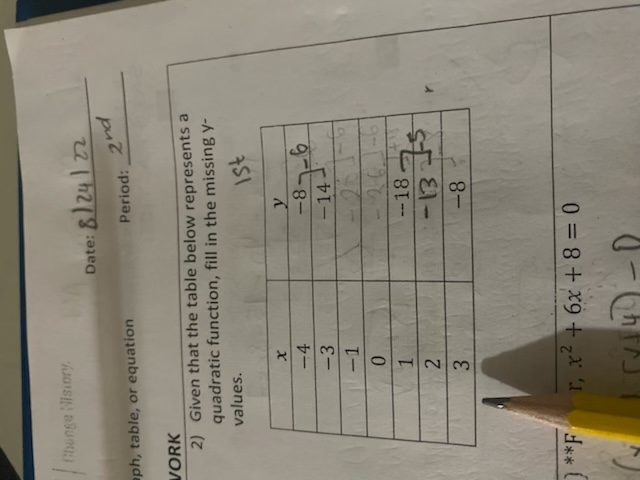elfmo20 2022-08-14

###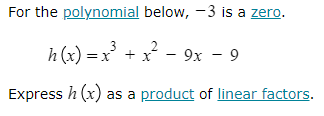Stephanie Wilkerson 2022-08-09

### Perform the indicated operations and indicate the degree of the resulting polynomial.(-8x^5+6x^2-9x+11)+(19x^5+3x^2-3x-14)betterthennewzv 2022-08-08

### Given f(x) = absoulte value of X and g(x)= x-2. Find $\left(f+g\right)\left(x\right),\left(f-g\right)\left(x\right),\left(fg\right)\left(x\right),\left(f/g\right)\left(x\right)$ and $\left(f\circ g\right)\left(x\right)$Bilgehan Yurtcan 2022-08-01

### can and how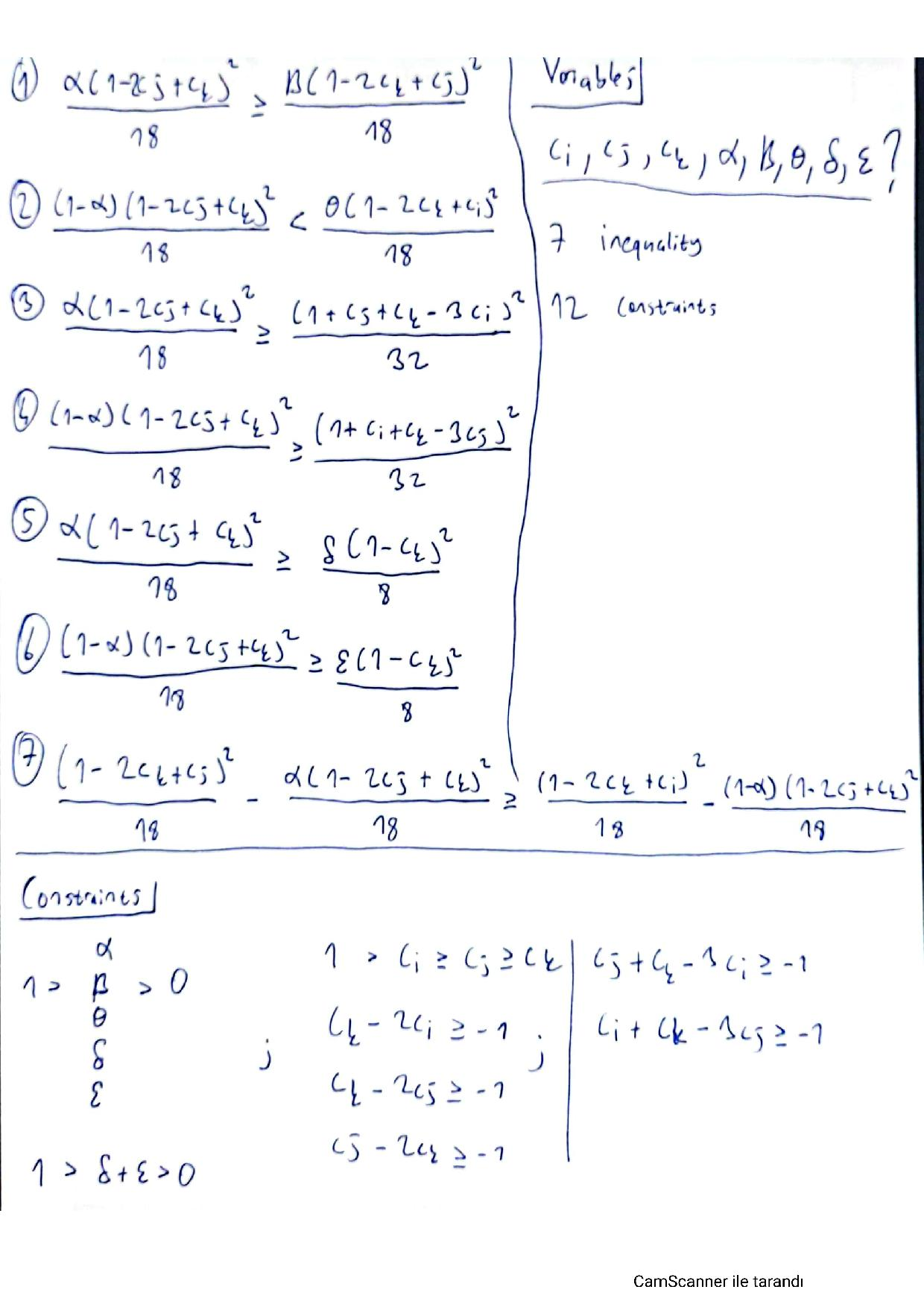you solve the set of inequality and find the intervals for variables in inequalities in the document which I uploaded using excel.Bilgehan Yurtcan 2022-07-31

### How can I solve set of inequality and find the intervals for variables in inequalities in the document which I uploaded using excel.Tyler Freeman 2022-07-21

### Which of the following options represents the same expression as (-3x)^2/3$\frac{1}{{\left(3x\right)}^{\frac{2}{3}}}$ (3x) -$\sqrt{{\left(3x\right)}^{2}}$ ${\left(\sqrt{-3x}\right)}^{2}$ $\sqrt{27{x}^{3}}$ $\sqrt{9{x}^{2}}$ ${\left(\sqrt{-3x}\right)}^{3}$Tyler Freeman 2022-07-21

### Which of the following options represents the same expression as (-3x)^2/3$\frac{1}{{\left(3x\right)}^{\frac{2}{3}}}$ (3x) -$\sqrt{{\left(3x\right)}^{2}}$ ${\left(\sqrt{-3x}\right)}^{2}$ $\sqrt{27{x}^{3}}$ $\sqrt{9{x}^{2}}$ ${\left(\sqrt{-3x}\right)}^{3}$Vicucyxycj 2022-07-17

###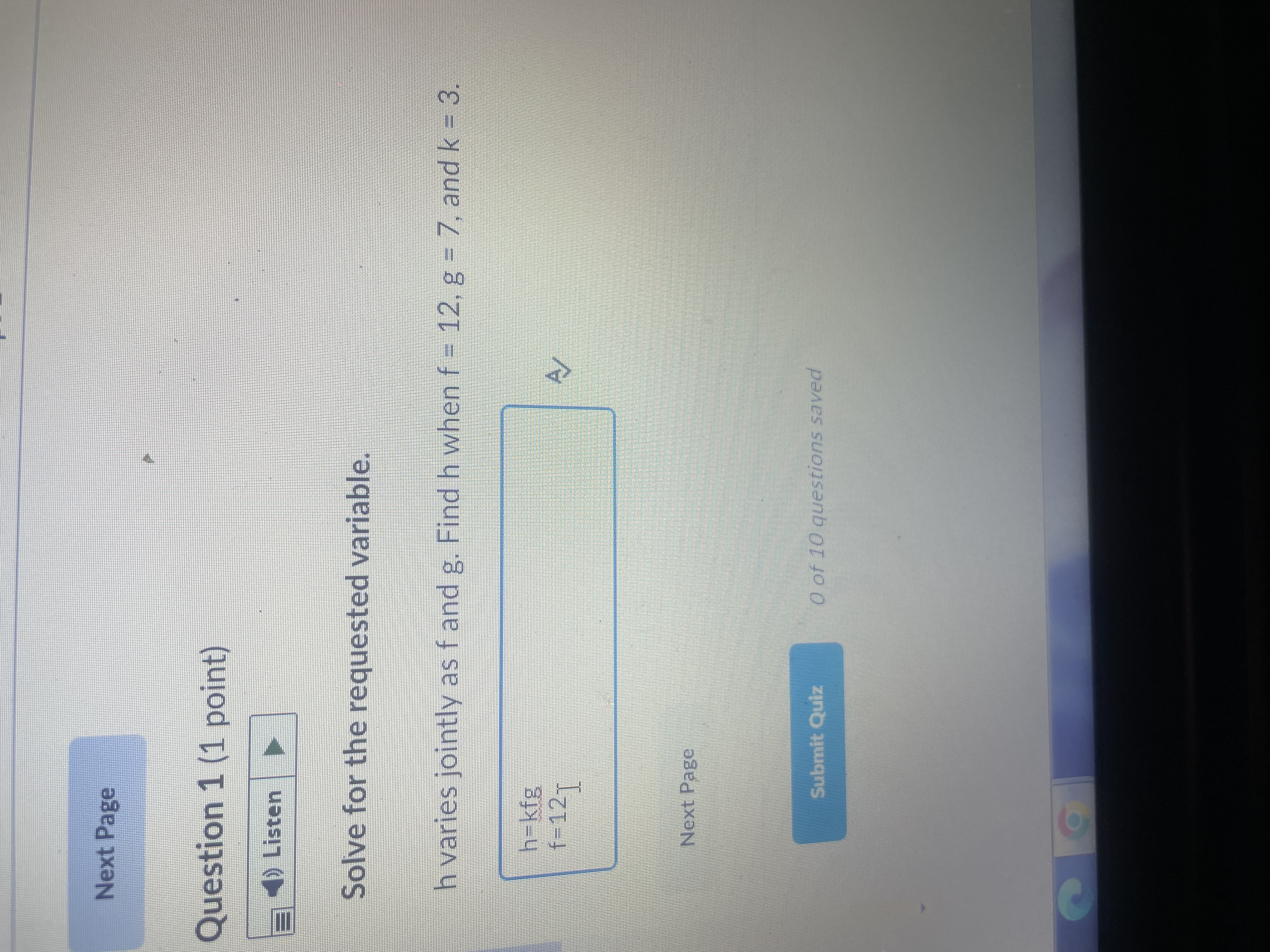Ismael Molina 2022-07-16

### Prove the identity$\mathrm{csc}x-\mathrm{cot}x\cdot \mathrm{cos}x=\mathrm{sin}x$Michelle Mendoza 2022-07-11

### I don't claim at all to be an expert on this topic. In many (advanced) linear algebra textbooks for undergraduates, I usually find something about the "Jordan Canonical Form" of a matrix.What is the purpose of such a form? I have taken a usual first-course in linear algebra (did another semester with Axler, but I don't claim to be an expert) and have taken abstract algebra (most familiar with group and ring theory) and have briefly skimmed through linear algebra books covering this material, but I don't quite understand the "big picture" idea, i.e., why is this useful in application? One person once told me it is the "most straightforward and useful algorithm for solving systems of linear equations, once you get beyond 3 variables or so," but maybe I'm missing something, since I usually don't see anything like what this person described to me in the linear algebra books I have. Most textbooks I've seen tend to have a more theoretical focus on this topic.Also, any suggested texts which have good coverage on this topic would be very helpful.esanchez0830 2022-07-08

### .               A group of students in MATH 105 start a walking program.  The chart below shows each member’s self-reported walking time in minutes during May 2022. X - 300 C - 400   F – 60A - 325  D - 900  G – 600                                               B - 550   E - 150   H – 250                                                 By what percent did X exceed or fall below the mean?ScommaMaruj 2022-07-08

### I am from a non-mathematics background but the course that I am taking in probability class is built on measure theory. I found many theorems stated in the book hard to comprehend and I think that's partly due to my insufficient maths background. I am self-learning real analysis currently and think it may be equally important to systematically learn abstract algebra if I really want to know the logic behind the probability class I am currently taking.I find myself completely lost proceeding to the following section of the text."Let $R$ be a relation, $A$ a mathematical object, and $x$ a letter (i.e., a "totally indeterminate" mathematical object). In the assembly of letters and fundamental signs which constitutes the relation $R$, replace the letter $x$ wherever it occurs by the assembly $A$. One of the criteria for forming relations is that the assembly so obtained is again a relation, which is denoted $\left(\ast \right)$ by the notation$\left(\mathrm{A}\mid x\right)\mathrm{R}$and is called the relation obtained by substituting $A$ for $x$ in $R$, or by giving $x$ the value $A$ in $R$. The mathematical object A is said to satisfy the relation $R$ if the relation $\left(\mathrm{A}\mid x\right)\mathrm{R}$ is true. It goes without saying that if the letter $x$ does not appear at all in the assembly $R$, then the relation $\left(\mathrm{A}\mid x\right)\mathrm{R}$ is just $R$, and in this case to say that $A$ satisfies $R$ means that $R$ is true."However, I do appreciate the textbook that is self-contained and appreciate the author devoted to mathematical reasoning so rigorously at the beginning of the chapter. I tried to find some textbook about mathematical logic but they are either too abstract or not thorough enough that seems to start from the most fundamental (i.e. from axiom and the most basic rule).I have read relevant posts on the subject I am asking but can't decide the material right for me. I am wondering if there are any materials or textbooks that introduce mathematical logic rigorously and serve as a supplementary text for me to understand the first chapter of the book? If there really isn't any textbook that is not too abstract but rigorous enough, I am wondering if there are any other textbooks on abstract algebra that start from mathematical logic and build the whole system from the scratch?Raul Walker 2022-07-04

### Often when I'm working on a math problem, there comes a point when I've set everything up and what remains is to expand some expression, substitute something in, solve an equation, or otherwise enter the domain of algebra.I find that I usually think about this step in the problem as a black box, into which I put a set of equations and out of which comes a set of solutions. This is particularly true if the algebra involved includes many steps.This is good in a lot of ways and bad in some others, but putting that aside, I'm fascinated that this step seems to come up in all areas of math. Is it possible to separate math from algebra? Are there any branches of math where long chains of algebra are not found or uncommon?Kaeden Hoffman 2022-07-04

### So, I finished my undergrad with a degree in applied mathematics, but when reading some graduate level texts and/or papers, I often find myself struggling. I eventually get there, but I often feel like I lack the intuition necessary to be able to come up with concepts on my own. I feel like I'm just missing some pivotal step in the journey to mathematical maturity.Does anyone have any advice?PS: If this means anything at all, as I was not a mathematics major, I did not take analysis or abstract algebra.Luis Rojas 2022-07-03

###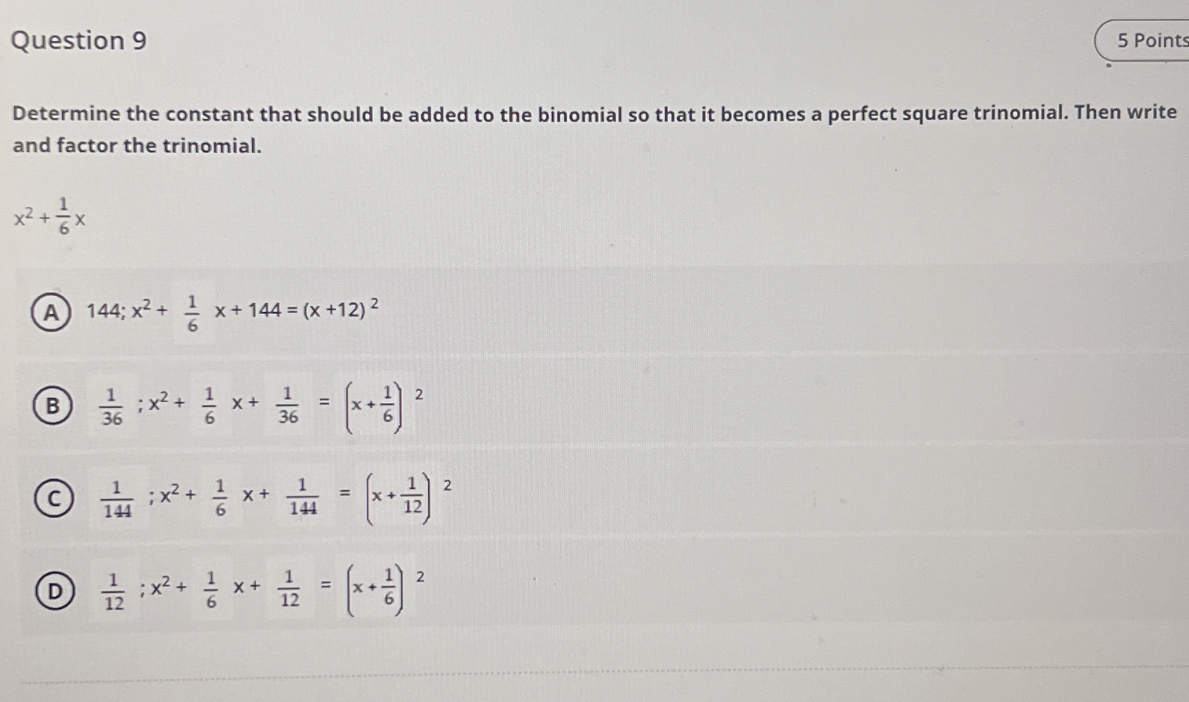Jovany Clayton 2022-07-03

### I am curious to know some theorems usually taught in advanced math courses which are considered 'generalizations' of theorems you learn in early university or late high school (or even late university).For example, I know that Stokes's theorem is a generalization of the divergence theorem, the fundamental theorem of calculus and Green's theorem, among I'm sure many other notions.I've read that pure mathematics is concerned mostly with the concept of 'generalization' and I am wondering which theorems/ideas/concepts, like Stokes's theorem, are currently celebrated 'generalizations' by mathematicians.

When you are getting enrolled in college, you still have to cope with all these college algebra problems and equations that often seem too challenging. When you are feeling confused, you should take a closer look at the questions that you have and seek the answers from what has been provided below. It will help you to start with the calculations and determine the most efficient solutions based on various methodologies. Make sure that you explore several college algebra examples because it will make it easier to compare and see the word problems as well when using the equations, too.